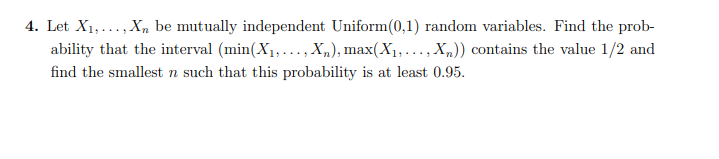Home / Answered Questions / Other / 4-let-x-x-be-mutually-independent-uniform-0-1-random-variables-find-the-prob-ability-that-the-interv-aw712

# (Solved): 4. Let X...... X Be Mutually Independent Uniform(0.1) Random Variables. Find The Prob- Ability That ...4. Let X...... X be mutually independent Uniform(0.1) random variables. Find the prob- ability that the interval (min(X1,...,xn), max(X1,...,xn) contains the value 1/2 and find the smallest n such that this probability is at least 0.95.

We have an Answer from Expert